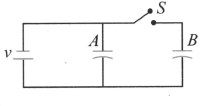Capacitors and capacitance
Question

# Figure shows two identical parallel plate capacitors connected to a battery through a switch S. Initially, the switch is closed so that the capacitors are completely charged. The switch is now opened and the free space between the plates of the capacitors is filled with a dielectric of dielectric constant 3. Find the ratio of the initial total energy stored in the capacitors to the final total energy storedDifficult
Solution

## Initial total energy $=\frac{1}{2}C{V}^{2}+\frac{1}{2}C{V}^{2}=C{V}^{2}$When the switch is open, dielectric is introduced. Then capacitance, C = KC = 3C. Energy stored in C is $=\frac{1}{2}3C{V}^{2}=\frac{3}{2}C{V}^{2}$Since the switch is open, charge will be the same in B so energy is $B=\frac{1}{2}\frac{C}{3}{V}^{2}$So total final energy $=\frac{3}{2}C{V}^{2}+\frac{1}{6}C{V}^{2}$$=\frac{9C{V}^{2}+1C{V}^{2}}{6}=\frac{10}{6}C{V}^{2}$So required ratio $=\frac{C{V}^{2}}{\frac{10}{6}C{V}^{2}}=\frac{3}{5}=3:5$

Get Instant Solutions